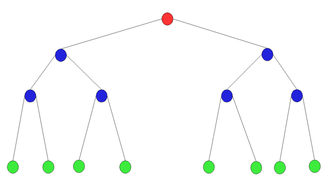# Build a Linked List For Each Layer in a Binary TreeI’ve been going through problems in Cracking the Coding Interview to keep my chops strong and for giggles and this one took a little bit of wrangling for me to get:

Given a binary search tree, design an algorithm which creates a linked list of all the nodes at each depth (i e , if you have a tree with depth D, you’ll have D linked lists)

So a binary tree such as :

```       (1)
/   \
/     \
(2)     (3)
/  \     / \
(4)  (5) (6) (7)
```

```(1) => NULL
(2) => (3) => NULL
(4) => (5) => (6) => (7) => NULL
```

I wrote up my solution to this in Python, and I’m going to share it with you to study and critique.

# Solution

If you’ve ever seen or written a linked list implementation before, you’ll probably realize there’s nothing particularly brilliant or innovative about this one. Just a good old-fashioned, simple singly linked list.

``````class LinkedList:
next = None
val = None

def __init__(self, val):
self.val = val

if self.next == None:
else:

def __str__(self):
return "({val}) ".format(val=self.val) + str(self.next)
``````

Usage:

``````ll = LinkedList(1)
``````

## The Binary Tree Implementation

The binary tree implementation is similarly from scratch, and simlarly simple.

``````class BinaryTree:
val = None
left = None
right = None

def __init__(self, val):
self.val = val

def __str__(self):
return "<Binary Tree (val is {val}). \n\tleft is {left} \n\tright is {right}>".format(val=self.val, left=self.left, right=self.right)
``````

No methods, I do all of the tree manipulation by hand. This works okay for problems of this (considerably small) scale.

## The Algorithm

The algorithm that I came up with is actually slightly different than what is listed as the solution in the book, and depends a bit of idiosyncracies of Python that aren’t in Java (which all of the solutions from the book are written in). Namely, it uses optional arguments to avoid wrapper methods and it uses a dictionary instead of a `ArrayList<LinkedList<BinaryTree>>`.

I also differ from the solution in the book in that I grab the depth of the tree once and use that to determine the linked list’s index, which is slightly less efficient than the solution that they provide. If I’m not mistaken, however, the asymptotic complexity is still the same (`O(n)`).

My depth function is exactly what you’d expect (recursive):

``````def depth(tree):
if tree == None:
return 0
if tree.left == None and tree.right == None:
return 1
else:
depthLeft = 1+depth(tree.left)
depthRight = 1+depth(tree.right)
if depthLeft > depthRight:
return depthLeft
else:
return depthRight
``````

My `tree_to_linked_lists` function does a pre-order traversal, adding nodes to their corresponding linked list (based on depth) in the dictionary `lists` as the tree is traversed. `lists` is passed into, and returned from (in its mutated state), each call to `tree_to_linked_lists`.

``````def tree_to_linked_lists(tree, lists={}, d=None):
if d == None:
d = depth(tree)
if lists.get(d) == None:
else:
if d == 1:
return lists
if tree.left != None:
if tree.right != None:
return lists
``````

This produces a result that is sort of in reverse order compared to the solution provided by the book, but it still satisfies the problem description to provide a collection of linked lists.

# Conclusion

You can find the entirety of the code here.

I need to be better at data structures and algorithms. They are fun.

Until next time, stay sassy Internet.

• Nathan
I want to help you become an elite engineer. Subscribe to follow my work with containers, observability, and languages like Go, Rust, and Python on Gumroad.

If you find a mistake or issue in this article, please fix it and submit a pull request on Github (must be signed in to your GitHub account).

I offer a bounty of one coffee, beer, or tea for each pull request that gets merged in. :) Make sure to cc @nathanleclaire in the PR.